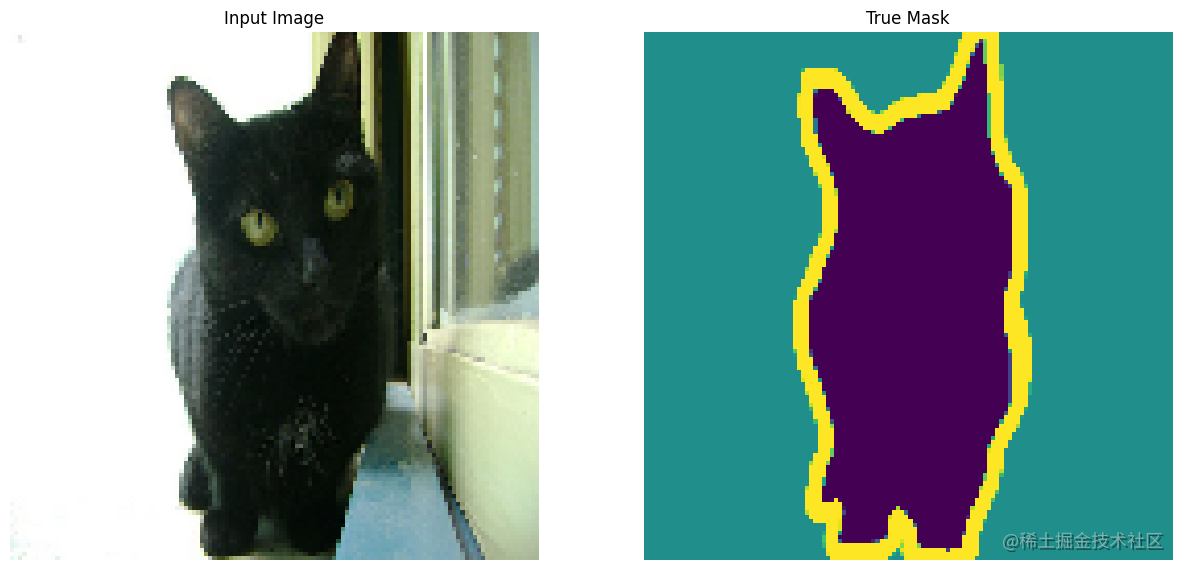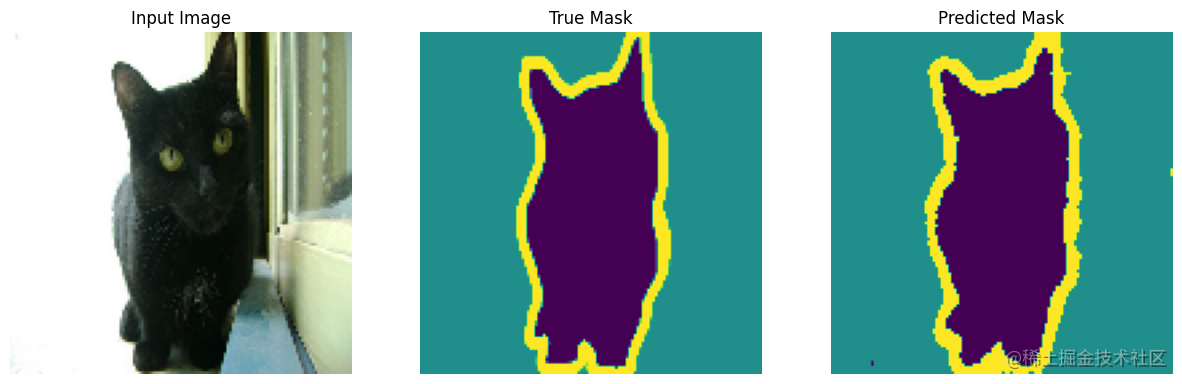# 准备

• pip install git+github.com/tensorflow/…
• pip install tensorflow == 2.10.1
• pip install tensorflow_datasets == 4.7.8
• pip install ipython == 8.6.0
• pip install matplotlib == 3.6.2

1. 获取数据
2. 处理数据
3. 搭建模型
4. 编译、训练模型
5. 预测

# 实现

### 1. 获取数据

（1）本文使用的数据集是 Oxford-IIIT Pet Dataset ,该数据集由 37 类宠物的图像组成，每个品种有 200 个图像（训练集和测试集各有 100 个），每个像素都会被划入以下三个类别之一：

• 属于宠物的像素
• 宠物边缘的像素
• 其他位置的像素

（2）可以使用 TensorFlow 的内置函数从网络上下载本次使用的数据 oxford_iiit_pet ，一般会下载到本地目录 ：C:\Users\【用户目录】\tensorflow_datasets\oxford_iiit_pet 。

（3）dataset 中存放是训练集和测试集这两个数据集，info 中存放的是该数据的基本信息，如文件大小，数据介绍等基本信息。

``````import tensorflow as tf
import tensorflow_datasets as tfds
from tensorflow_examples.models.pix2pix import pix2pix
from IPython.display import clear_output
import matplotlib.pyplot as plt

### 2. 处理数据

（1）normalize 函数主要是完成将图像颜色值被归一化到 [0,1] 范围，掩码像素的所属标签被标记为 {1, 2, 3}。为了方便后面的模型计算，将它们分别减去 1，得到的标签为：{0, 1, 2} 。

（3）从 dataset 中分理处训练集 train_images 和测试集 test_images 。

``````def normalize(input_image, input_mask):
input_image = tf.cast(input_image, tf.float32) / 255.0

input_image = tf.image.resize(image['image'], (128, 128))

（4）为了保证在加载数据的时候不会出现 I/O 不会阻塞，我们在从磁盘加载完数据之后，使用 cache 会将数据保存在内存中，确保在训练模型过程中数据的获取不会成为训练速度的瓶颈。如果说要保存的数据量太大，可以使用 cache 创建磁盘缓存提高数据的读取效率。另外我们还使用 prefetch 在训练过程中可以并行执行数据的预获取。

``````TRAIN_LENGTH = info.splits['train'].num_examples
BATCH_SIZE = 32
BUFFER_SIZE = 1000
train_batches = (train_images.cache().shuffle(BUFFER_SIZE).batch(BATCH_SIZE).repeat().prefetch(buffer_size=tf.data.AUTOTUNE))
test_batches = test_images.batch(BATCH_SIZE)

（5）这里的 display 函数主要是将每个样本的宠物图像、对应的掩码图像、预测的掩码图像绘制出来，在这里我们只随机挑选了一个样本进行显示。因为这里还没有预测的掩码图像，所以没有将其绘制出来。

（6）我们可以看到左侧是一张宠物的生活照，右边是一张该宠物在照片中的轮廓线图，宠物的样子所处的像素为紫色，宠物的轮廓边缘线的像素是黄色，背景的像素是墨绿色，这其实对应了图片中的像素会分成三个类别。

``````def display(display_list):
plt.figure(figsize=(15, 15))
for i in range(len(display_list)):
plt.subplot(1, len(display_list), i+1)
plt.title(title[i])
plt.imshow(tf.keras.utils.array_to_img(display_list[i]))
plt.axis('off')
plt.show()### 3. 搭建模型

（1）这里使用的模型是修改后的 U-Net ，详细内容可看链接。U-Net 由编码器（下采样器）和解码器（上采样器）组成。为了学习稳健的特征并减少可训练参数的数量，请使用预训练模型 MobileNetV2 作为编码器。对于解码器，您将使用上采样块，该块已在 TensorFlow Examples 仓库的 pix2pix 示例中实现。

（2）如前所述，编码器是一个预训练的 MobileNetV2 模型。您将使用来自 tf.keras.applications 的模型。编码器由模型中中间层的特定输出组成。请注意，在训练过程中不会训练编码器。

（3）我们这里使用模型由两部分组成， 一个是编码器 down_stack（也就是下采样器），另一个是解码器 up_stack （也就是上采样器）。我们这里使用预训练的模型 MobileNetV2 作为编码器， MobileNetV2 模型可以直接从网络上下载到本地使用，使用它来进行图片的特征抽取，需要注意的是我们这里选取了模型中的若干中间层，将其作为模型的输出，而且在训练过程中我们设置了不会去训练编码器模型中的权重。对于解码器，我们使用已经在仓库实现了的 pix2pix 。

（4）我们的 U-Net 网络接收的每张图片大小为 [128, 128, 3] ，先通过模型进行下采样，然后计算上采样和 skip 的特征连接，最后经过一层 Conv2DTranspose 输出一个大小为 [batch_size, 128, 128, 3] 的向量结果。

``````base_model = tf.keras.applications.MobileNetV2(input_shape=[128, 128, 3], include_top=False)
layer_names = [ 'block_1_expand_relu', 'block_3_expand_relu', 'block_6_expand_relu', 'block_13_expand_relu', 'block_16_project']
base_model_outputs = [base_model.get_layer(name).output for name in layer_names]
down_stack = tf.keras.Model(inputs=base_model.input, outputs=base_model_outputs)
down_stack.trainable = False
up_stack = [  pix2pix.upsample(512, 3),  pix2pix.upsample(256, 3),   pix2pix.upsample(128, 3),   pix2pix.upsample(64, 3)]

def unet_model(output_channels:int):
inputs = tf.keras.layers.Input(shape=[128, 128, 3])
skips = down_stack(inputs)
x = skips[-1]
skips = reversed(skips[:-1])
for up, skip in zip(up_stack, skips):
x = up(x)
concat = tf.keras.layers.Concatenate()
x = concat([x, skip])
last = tf.keras.layers.Conv2DTranspose( filters=output_channels, kernel_size=3, strides=2, padding='same')
x = last(x)
return tf.keras.Model(inputs=inputs, outputs=x)

### 4. 编译、训练模型

（1）因为每个像素面临的是一个多类分类问题，所以我们使用 SparseCategoricalCrossentropy 作为损失函数，计算多分类问题的交叉熵，并将 from_logits 参数设置为 True，因为标签是用 0、1、2 三个整数表示。SparseCategoricalCrossentropy 函数中当 from_logits=true 时，会先对预测值进行 Softmax 概率化，就无须在模型最后添加 Softmax 层，我们只需要使用经过 Softmax 输出的小数和真实整数标签来计算损失即可。reduction 默认设置为 auto 时，会对一个 batch 的样本损失值求平均。

``````y_true = [0,1,2]
y_pred = [[0.2,0.5,0.3],[0.6,0.1,0.3],[0.4,0.4,0.2]]

loss_fn = tf.keras.losses.SparseCategoricalCrossentropy(from_logits=False,name='sparse_categorical_crossentropy')
loss_val = loss_fn(y_true,y_pred).numpy()
loss_val
1.840487

(-np.log(0.2)-np.log(0.1)-np.log(0.2))/3
1.8404869726207487

``````OUTPUT_CLASSES = 3
EPOCHS = 20
VAL_SUBSPLITS = 5
STEPS_PER_EPOCH = TRAIN_LENGTH // BATCH_SIZE
VALIDATION_STEPS = info.splits['test'].num_examples//BATCH_SIZE//VAL_SUBSPLITS

model = unet_model(output_channels=OUTPUT_CLASSES)
model_history = model.fit(train_batches, epochs=EPOCHS, steps_per_epoch=STEPS_PER_EPOCH, validation_steps=VALIDATION_STEPS, validation_data=test_batches)

``````115/115 [==============================] - 110s 961ms/step - loss: 0.1126 - accuracy: 0.9473 - val_loss: 0.3694 - val_accuracy: 0.8897

### 5. 预测

（1）使用 create_mask 我们会将对该批次的第一张图片的预测掩码图像进行展示，结果是一个大小为 (128, 128, 1) 的向量，其实就是给出了该图片每个像素点的预测标签。

（2）在这里我们使用了上面的一个样本 sample_image ，使用训练好的模型进行预测，因为这里的样本 sample_image 是的大小是 (128, 128, 3) ，我们的模型需要加入 batch_size 维度，所以在第一维扩展了一个维度，大小变为 (1, 128, 128, 3) 才能输入模型。

（3）从绘制的预测掩码图像结果看，预测宠物边界线已经相当清晰了，如果进一步调整模型结果和训练的迭代次数，效果会更加好。

``````def create_mask(pred_mask):